# Perl – Listing your Program with a Debugger

Perfect programs are hard to get in the very first attempt. They have to go through various steps of debugging to fix all errors. There are two types of errors – Syntax errors and Logical errors. Syntax errors are easy to fix and are found fast. On the other hand, logical errors are hard to find and fix. Thus, a debugger is required to fix those errors.

In Perl, a debugger is an environment that executes the program line by line. This process is also known as single-stepping through the program. To enter into debugger, follow the Syntax given below:

Syntax:

`perl -d <program_name>`

Sample Program to Debug:

 `#!/usr/bin/perl -w ` ` `  `# Perl program for a simple calculator ` `use` `strict; ` `     `  `my` `\$op``; ` `my` `\$num1``; ` `my` `\$num2``; ` `my` `\$result``; ` `my` `\$flag``; ` `     `  `calculator(); ` `     `  `sub` `calculator  ` `{ ` `    ``print` `"Enter operation you want to perform -Add, Sub, Mult, Div - "``; ` `    ``chomp``(``\$op` `= <>); ` `    ``print` `"Enter first number: "``;``chomp``(``\$num1` `= <>); ` `    ``print` `"Enter second number: "``;``chomp``(``\$num2` `= <>); ` ` `  `    ``# Check for arithmetic operation ` `    ``if` `(``\$op` `=~ /^a/) { ` `        ``\$result` `= ``\$num1` `+ ``\$num2``; ` `    ``} ``elsif` `(``\$op` `=~ /^s/) { ` `        ``\$result` `= ``\$num1` `- ``\$num2``; ` `    ``} ``elsif` `(``\$op` `=~ /^m/) { ` `        ``\$result` `= ``\$num1` `* ``\$num2``; ` `    ``} ``elsif` `(``\$op` `=~ /^d/) { ` `        ``\$result` `= ``\$num1` `/ ``\$num2``; ` `    ``} ` ` `  `    ``# Print the answer of above operation ` `    ``print` `"Result: \$result\n"``; ` ` `  `    ``# Calling the function recursively ` `    ``print` `"Do another calculation ? "``;``chomp``(``\$flag` `= <>); ` `    ``if` `(``\$flag` `=~ /^y/) { ` `        ``calculator(); ` `    ``} ``else` `{ ` `        ``print` `"Thank You !!\n"``; ` `    ``} ` `} `

#### Listing Sample Code with Debugger

1. ‘l’ command :
The ‘l’ command lets us print a partial part of our scripts. There are several versions of this command that we can use –

• Use ‘l’ – Displays 10 lines of script from location of cursor.
• Using l 4+6 – Displays 6 lines of script starting from line 4.
• Using l 4-7 – Displays lines 4 through 7 of script.
• Using l 20 – Displays script on line 20.
• Using l foo – Displays approximately first 10 lines of foo() function.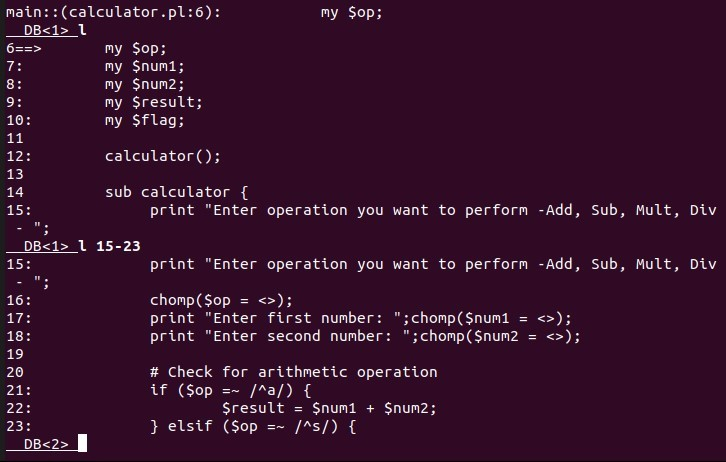2. ‘-‘ command :
Outputs 10 lines of script before the current line. Suppose that you are current on line 20, then, lines 9 to 19 will be displayed.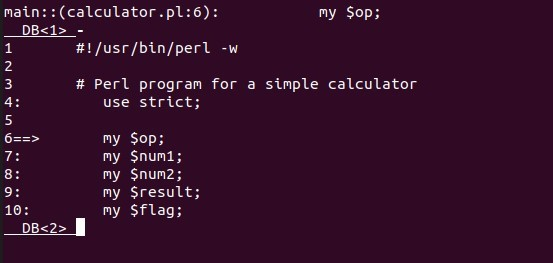3. ‘w’ command :
Syntax:

`w \$variable_name`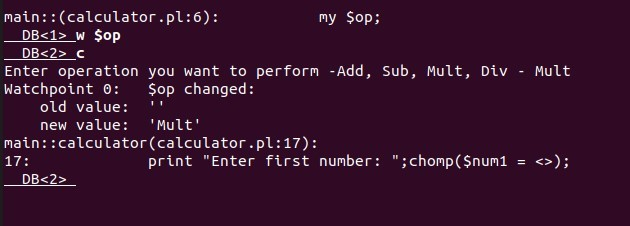4. ‘//’ and ‘??’ :
// and ?? search for a given pattern in the script. The /pattern/ searches for a pattern in forward direction while, the ?pattern? searches for a pattern in the backward direction from the current position of cursor.
Syntax:

`/pattern/ or ?pattern?`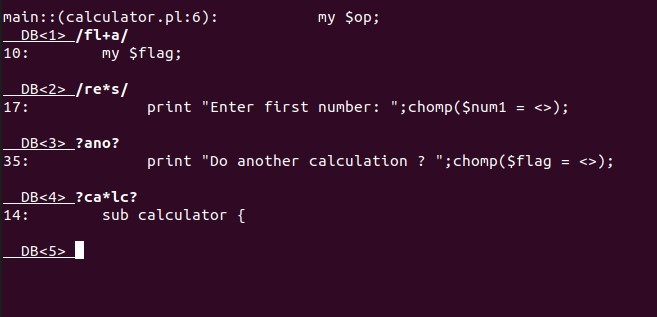5. ‘S’ command :
This command lists all the subroutines not matching a given pattern.
Syntax:

`S expression `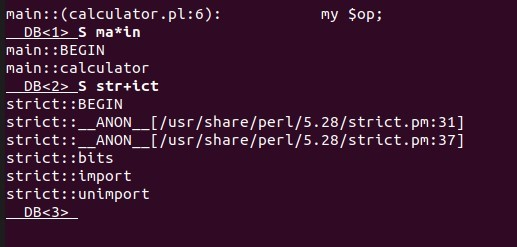My Personal Notes arrow_drop_upCheck out this Author's contributed articles.

If you like GeeksforGeeks and would like to contribute, you can also write an article using contribute.geeksforgeeks.org or mail your article to contribute@geeksforgeeks.org. See your article appearing on the GeeksforGeeks main page and help other Geeks.

Please Improve this article if you find anything incorrect by clicking on the "Improve Article" button below.

Article Tags :
Practice Tags :

Be the First to upvote.

Please write to us at contribute@geeksforgeeks.org to report any issue with the above content.